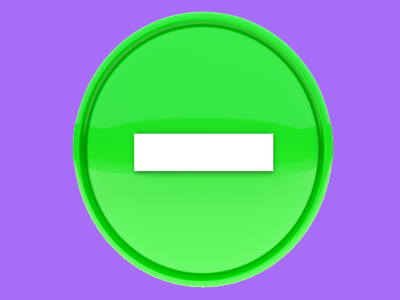Difference, subtract, subtraction and minus are all words which mean take away.

# Year 2 Calculation - Subtraction Language

This quiz addresses the requirements of the National Curriculum KS1 Maths and Numeracy for children aged 6 and 7 in year 2. Specifically this quiz is aimed at the section dealing with understanding the language of subtraction.

In Year 2, children extend their understanding of the language of subtraction to include 'difference'. They should already know and be using words such as subtract, subtraction, take away and minus. Recognising the language of subtraction is essential in order to be able to make decisions about which operation to use to solve calculations and problems.

Question 1
I have 15 cakes and eat 6. How many are left?
6
9
8
4
'How many left' is a clue that this is a subtraction
Question 2
What is the difference between 12 and 5?
One is odd, one is even
7
One is 2-digit, the other isn't
17
The 1st and 3rd options are differences but in maths 'difference' means subtract. To find the difference, subtract the smaller number from the larger one
Question 3
Which of the following is not a subtraction word?
Take away
Subtract
Multiply
Minus
Multiply does not mean subtract
Question 4
What is the symbol for subtraction?
-
x
+
=
This sign means to subtract or take away
Question 5
8 is the difference between which 2 numbers?
2 and 4
12 and 20
6 and 10
12 and 14
To find the difference, subtract the smaller number from the larger one
Question 6
What is 16 take away 6?
16
6
26
10
'Take away' is another way of saying subtract
Question 7
How much is 6 less than 18?
12
8
9
15
'Less than' is a subtraction word - you could also count backwards
Question 8
17 minus which number = 5?
7
12
5
9
Minus means to take away. 17 - 12 = 5
Question 9
Which word could be used in this number sentence?
12 ___ 5 = 7
subtract
plus
total
Subtract means to take away
Question 10
Which word could be used in this number sentence?
15 ___ 9 = 6
plus
divided by
minus
Minus means to subtract